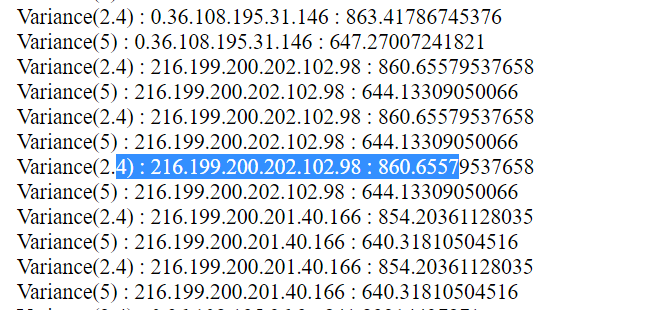# Variance for multiple values

``````          <html><body>
<?php

\$counte =0;\$i = 0;
\$file = fopen("SIS.txt","r");
while(! feof(\$file))
{
\$b[\$i] = trim(fgets(\$file));
\$i++;
} \$count1 = count(\$b);
for(\$j=0;\$j<\$count1;\$j++){
\$file_handle = fopen("results.csv", "r");
while ( \$line_of_text = fgetcsv(\$file_handle)){
if (\$line_of_text == \$b[\$j]) {
\$counte++;
\$van1[] = \$line_of_text;
\$van2[] = \$line_of_text;
}
}\$counte=0;
}
fclose(\$file_handle);
\$fMean1 = array_sum(\$van1) / count(\$van1);
\$fMean2 = array_sum(\$van2) / count(\$van2);
\$variance1 = array_sum(array_map(function (\$x1) use (\$fMean1) {
return pow(\$x1 - \$fMean1, 2);
}, \$van1)) / count(\$van1);
echo "Variance(2.4) : ".  \$variance1. "<br/>";
\$variance2 = array_sum(array_map(function (\$x2) use (\$fMean2) {
return pow(\$x2 - \$fMean2, 2);
}, \$van2)) / count(\$van2);
echo "Variance(5) : ". \$variance2;
?>
``````

Is there a question here?

1 Like

I want to take variance for multiple values, I’m just getting variance for single value. if (\$line_of_text == \$b[\$j]) in this condition when i want to find variance for \$b,\$b,\$b,… but as of now i’m just getting variance for last value.

I think the reason is that you’re doing the calculations outside of the loop that iterates through the `\$b` array - if you indented the code more consistently it would be easier to check.

If that’s the case, move the code inside the loop and it should do the calculations for each value of `\$j`.

Now it is working but some values it is repeating twice or thrice.boxplot.php (918 Bytes)

As your variance is based on the average of the values on the `\$van` array, don’t you need to clear that array each time you change the value of `\$j`? I’m not sure what the maths is doing, but it doesn’t seem correct that when you run through matches for `\$b` for example, you also include the values for `\$b` and `\$b` because they’re still in `\$van`.

No there is no pattern, for mean i need an array if i’ll write inside loop it’ll take a single value so outside it is calculating an array for mean. it is now repeating for all values just a few are there which are repeating. i mean out of 70 it is repeatin 15 values twice or thrice others are only one time

I take it you’ve checked the obvious, that there’s no duplication in the SIS file? var_dump the `\$b` array after you’ve built it in the first section of code, to check.

No there is no duplication i checked repeating values already but didn’t get any duplication.

Hmm, strange. I can’t see anything obvious. Can you post the two file to run the code, the SIS and the CSV?

Sure. results.csv (175.8 KB)
SIS.txt (1.5 KB)

Ah, there’s the problem. You set the value of `\$val` inside the if() clause while you are searching the array for entries from the CSV file. But, you don’t check whether you found any entries. So what appears to be duplicate entries are not - if you use the \$counte variable properly, surround your output with a check to see whether that’s greater than zero, and reset it for each value of `\$j`, it should work OK.

So in your example, what appears to be the second display for 216.199.200.202.102.98 is actually the display for the next value in the SIS file / \$b array, which has no entries in the CSV file. Because you’ve set \$val for the previous loop, and don’t check your count, you calculate and display incorrect values.

Actually i have like 14-24 values for each ap so if few values are zero it will not impact to variance because will work with other values of that ap.

What I meant was, because there are values in the \$b array that are not present in the CSV file, you are displaying the information from the previous value, which presents as a duplicate because it retained the variables from last time around the loop.

216.199.200.202.102.98 has 16 entries in the csv file, displays valid data for that number
216.199.200.201.40.193 is the next, and has zero entries in the csv file, but it displays the previous values, including the long number.

oh is it. Ok will add one for more condition for that. Thanks

Going off-topic perhaps, but you may want to condsider adding a statistics extension. It would require some work to install it but if you are going to be doing a lot of statistics math it could be worth the effort.

http://php.net/manual/en/book.stats.php

i tried with if(\$b[\$j]>0) afew more but not working tried with else too and already did reset counter.

That won’t do it - that’s the array that contains your numbers like 202.196.200.1.4 or similar. You need to be looking at the value of `\$counte` - that’s your counter for how many of each value are found.

If that doesn’t help, paste the current version of the code in here and we’ll see what might be causing it. Show where you’re resetting the counter, it’s not in the code you link to in post #6.

Here you can check - boxplot.php (994 Bytes)

You’re resetting the `\$counte` counter to zero inside the `while()` loop, which means you can’t use it to check whether you got any results. In fact, it’s hardly worth having the counter if you’re going to zero it every time you read another value from the CSV file.

Move that line to after all your calculations where you display the variance, and surround those calculations and the display with `if (\$counte > 0)` and you should find it only displays information where the string is found in the csv file.